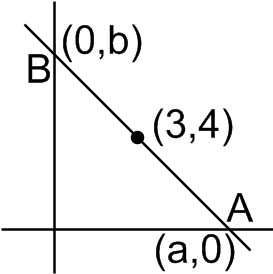Q

# Tell me? - Co-ordinate geometry - JEE Main-5

A straight line through the point is such that its intercept between the axes is bisected at . Its equation is,

• Option 1)

• Option 2)

• Option 3)

• Option 4)

122 Views

As we learnt in

Intercept form of a straight line -

- wherein

and are the -intercept and -intercept respectively.a = 6

b = 8

Option 1)

This is incorrect option

Option 2)

This is incorrect option

Option 3)

This is correct option

Option 4)

This is incorrect option

Exams
Articles
Questions